# Transit Equilibrium Assignment based on Optimal Strategies: An Implementation in EMME/2

Heinz Spiess
EMME/2 Support Center, Haldenstrasse 16, CH-2558 Aegerten, Switzerland
`heinz@spiess.ch`

June 1993

### Abstract:

The standard transit assignment offered in EMME/2, which is based on optimal strategies, does not consider congestion effects due to limited vehicle capacity. This assignment model can be extended by taking into account the vehicle capacity by means of a volume-dependent transit time function, leading to the formulation of a transit equilibrium assignment model. In this note, we describe how the standard version of the EMME/2 Transportation Planning Software can be used to solve this assignment model. A macro has been written which implements a Frank-Wolfe descent algorithm, by combining the fixed cost transit assignment module with the network and matrix calculator modules.

# Introduction

In most transit assignment applications, congestion effects due to overcrowding of the vehicles are not taken into account for modeling of the route choice. This is a reasonable approach in all those cases where the goal of the planning process is to provide enough capacity for all transit passenger on the routes of their choice. There are, however, situations in which it is not feasible to provide enough transit capacity to preclude congestion. In these cases, the route choice of the transit passenger is likely to be influenced by the congestion on board the vehicles, so that some travelers will switch from congested to less congested routes, even if the latter are less attractive in terms of travel time or cost.

In this note, we describe an implementation of an equilibrium transit assignment based on the concept of optimal strategies. The congestion is modeled by means of volume dependent cost functions, similar to the volume-delay functions used in the highway equilibrium assignment. After having presented the mathematical formulation of the model, we discuss its implementation in EMME/2.

# Fixed Cost Assignment Model

In this section we briefly describe the fixed cost transit assignment model based on optimal strategies. For a more detailed description of the model, as well as the proofs, refer to Spiess (1984) and Spiess and Florian (1989).

For the sake of easier presentation of the mathematical formulation of the model, the transit network is assumed to be represented by a standard node/link type network, where a set of nodes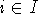is connected by a set of links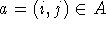. The set of links going out of node i (forward star) is denoted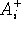, and the set of incoming links (backward star) is denoted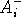.

A travel time (or cost)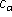and a service frequency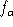is associated with each network link a. The demand between nodes i and j is given by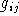.

Note that in this type of ``exploded'' network representation, the itineraries of the transit lines are implicitly contained in the network topology. The set of nodes not only contains the physical nodes of the underlying street or rail network, but also one additional node for each transit stop of each line. Correspondingly, the links are subdivided into various classes, such as boarding, alighting, in-vehicle and walking links. Note that only boarding links imply waiting, thus have a finite frequency. All other links are served continuously (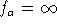).

The waiting time at a node depends on the set of attractive links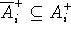, i.e. the set of outgoing links which are considered for travel by the travelers by boarding the first vehicle leaving on any of these links. For any given set of attractive links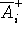at node i, the combined waiting time is proportional to the combined total frequency of these links is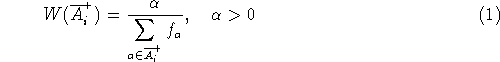and the probability of leaving node i on link a isGiven the above relations, any strategy for reaching destination r is completely defined by the corresponding subset of attractive links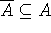.

The optimal strategy for reaching a destination is the one which minimizes the total expected cost. Note that the cost of a strategy is the sum of link travel timesweighted by the probability of traveling on link a, and the waiting time at nodes i weighted by the probability of traveling through node i. It has been shown that for fixed link travel times, the assigning of the trips from all origins to destination r according to the optimal strategy corresponds to solving the following linear optimization problem: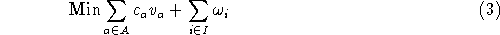subject to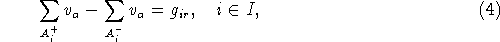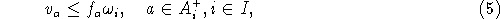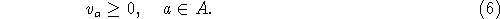Note that the variables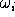represent the total waiting time (in person minutes) at node i.

The problem (3) can be solved very efficiently by means of the following label-setting type algorithm: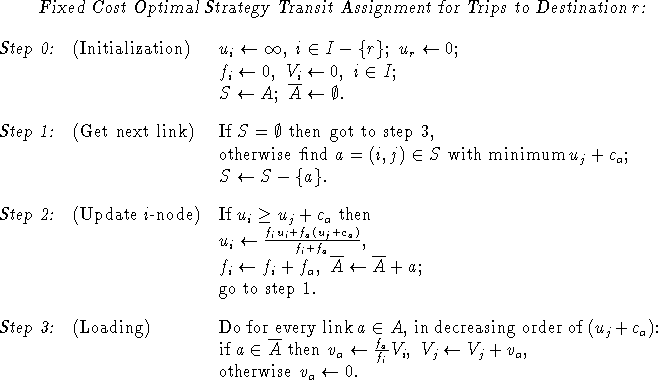# Transit Assignment with Non-Linear Cost Functions

We now turn our attention to the the variant of the transit assignment problem in which the link travel timesare no longer constants, but are continuous non-decreasing functions of the corresponding link flows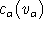. Such a dependence of the link cost on the transit volume may represent an actual slowing down of the transit vehicle due to the number of passengers, but it may also be interpreted as a generalize cost which includes a ``discomfort'' term which increases as the vehicles get crowded.

In this context, the transit assignment problem is no longer separable by destination node, since the link costs depend on the total flow of passengers. The total transit volumes are the sum of the volumes bound for each of the destinations.

As the expected cost of any given strategy is no longer fixed, but depends on the total volumes, the optimal strategies are now defined by Wardrop's second principle, which implies that only strategies with minimal expected cost will be used by the travelers (Wardrop, 1952). The resulting equilibrium assignment is equivalent to the following convex minimization problem: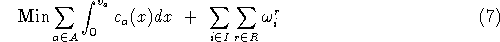subject to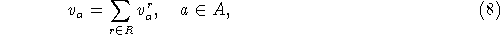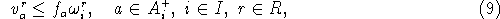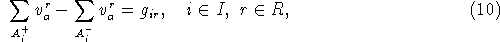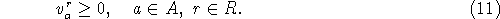As has been shown by Spiess (1984), the above problem can be solved by applying the successive linear approximation method (Frank and Wolfe, 1956). An important advantage of this method is the fact that only total volumes need to be computed and stored, since the destination dependent volumes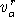are dealt with implicitly.

Optimal Strategy Equilibrium Transit Assignment:

 Step 0: (Initialization)Step 1: (Subproblem)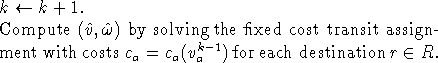Step 2: (Line Search)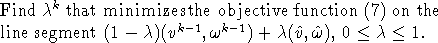Step 3: (Update)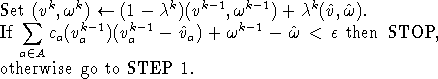The minimization in Step 2 is best implemented not by actual minimization, but by annulling the derivative, i.e. solving the equation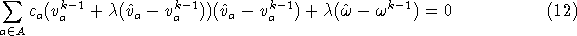Note that the stopping criterion used in Step 3 of the above algorithm corresponds to the absolute gap, which is an upper bound for the difference between the objective function at the current solution and at the true optimum.

# Implementation in EMME/2

In this section we describe how the transit equilibrium assignment can be implemented in the EMME/2 Transportation Planning Software (Spiess, 1984, and INRO, 1992). An important feature of EMME/2 is its modularity. The various functionalities used in the transportation planning practice are implemented as a set of independent basic tools, all acting on a common data bank. These can easily be used individually or in combination to form more complex models. A powerful macro language is provided within the EMME/2 system, which allows the user to implement the various steps of the model and to automate the procedure.

To implement the equilibrium transit assignment discussed in the previous section, the following basic EMME/2 tools are used:

• Fixed cost transit assignment (Modules 5.11/5.31): This is the standard EMME/2 transit assignment model. It implements the optimal strategy assignment described earlier. Of course, in EMME/2 the transit network is expressed by explicit transit line itineraries, so that the user need not be concerned with the ``exploded'' network representation used here for the mathematical formulation of the model. The travel cost on each transit line segment is given by applying the corresponding user definable travel time function. The assignment can be finetuned by various parameters and weights, which are not discussed here.
• Network Calculator (Module 2.41): This is a very general tool to evaluate algebraic expressions combining any kind of network information. Automatic conversion between the different element levels (node, link, transit line, transit segment) is provided.
• Matrix Calculator (Module 3.21): Similar to the Network Calculator, this tool allows the evaluation of expressions containing any kind of matrix information. Automatic conversion between the different matrix formats (full matrix, origin vector, destination vector, scalar value) is provided.

The travel cost functionis given by a fixed travel time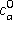and a volume dependent congestion function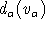in the form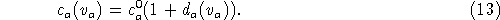The congestion function can be any non-decreasing function with d(0)=0. It models the discomfort of traveling on a segment at a volume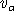. Since the function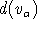is specified as a network calculator expression, it can access any other attribute of the transit line as well, such as: headway, seated and total vehicle capacity, user attributes. By default, BPR-type and conical congestion functions are provided (Spiess, 1990), but the macro allows easy integration of other functional forms that might be required for particular applications.

The fixed travel costs are, as usual, coded directly into the transit time functions. In order to enable the transit time functions to reflect congestion costs, all transit time functions have to be multiplied with the term `*(1+US1)`. During the assignment steps, the user defined segment attribute `US1` will contain the value of the congestion function.

In terms of the so defined congestion function, the objective function of the equilibrium assignment (7) separates in a (linear) travel time part T and a (non-linear) congestion partThe derivative of the objective function with respect to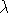(12), used to compute the optimal step length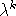, is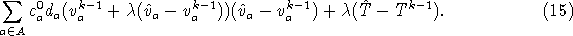With the above preliminaries, we can now outline the implementation of the EMME/2 equilibrium transit assignment macro CONGTRAS (CONGested TRansit ASsignment):

 Step: Description: Module: 1 Initialize congestion costs `US1` to 0. 2.41 2 Compute total number of transit trips and initialize iteration counter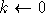. 3.21 3 Perform uncongested fixed cost assignment to obtain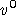and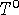. 5.11/5.31 4 Compute congestion cost . 2.41 5 Increment iteration counter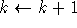. 3.21 6 Compute new segment congestion costs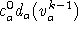into `US1`. 2.41 7 Perform fixed cost transit assignment with new congestion costs to obtainand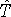. 5.11/5.31 8 Compute stopping criterion `GAP`. 2.41 9 Perform line search for obtaining optimal step length. This is implemented using the secant method to annul (15). 2.41 10 Update transit volumes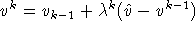. 2.41 11 Update total travel time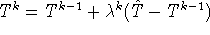. 3.21 12 Test normalized gap stopping criterion. If `GAP`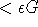then STOP, else continue with step 5. 2.41

For technical reasons, this step is implemented in the macro not in module 2.41, but using low level data manipulations in module 1.11.

In its current form, the CONGTRAS macro only considers crowding within the transit vehicles. But of course, as can be seen from the model formulation in the previous section, it is also possible to include congestion discomfort outside the transit vehicles, such as crowding on the platforms and on the pedestrian walk link - as long as the discomfort function is symmetric (i.e. the same travelers that are causing the congestion are also suffering the effects of it).

# Conclusions

We have shown that it is possible to implement a true equilibrium transit assignment within the framework of the standard EMME/2 system. A variant of the macro outlined above is being used at London Transport for modeling crowding in the London Underground. Instead of using one of the default congestion functions which are based on nominal capacity, the macro has been modified for taking into account the actual profile of train density and passenger load during peak period. The details of this project are described in Abraham and Kavanagh (1992).

It can be argued with good reason, that the modeling congestion should be done using asymmetric congestion functions, e.g. as the perceived frequency of a line for a boarding passenger depending on the number of passengers already on board, or the dwell time of a line at a node depending on the number of boarding and alighting passengers. While it is indisputable that such phenomena occur in reality, including them into assignment models as the one described here unfortunately leads to models with non-unique solutions. Since the uniqueness of the solution is a primordial requirement for any assignment model, such asymmetric models, even those for which convergent algorithms are available, are of very limited practical use.

## References

1
Abraham H. and Kavanagh C. (1992). Modelling Public Transport In-vehicle Congestion Using EMME/2 Release 5. Paper presented at the 1st European EMME/2 Users Conference, London, April 1992.

2
Frank M. and Wolfe P. (1956). An algorithm for quadratic programming. Nav. Res. Logist. Q. 3, 95-110.

3
INRO Consultants Inc. (1992). EMME/2 User's Manual.

4
Spiess H. (1984). Contributions à la théorie et aux outils de planification de réseaux de transport urbain. Ph.D. thesis, Département d'informatique et de recherche opérationnelle, Centre de recherche sur les transports, Université de Montréal, Publication 382.

5
Spiess H. (1990). Conical Volume-Delay Functions. Transportation Science 24, No. 2, 153-158.

6
Spiess H. and Florian M. (1989). Optimal strategies: A new assignment model for transit networks Transpn. Res. B 23, 83-102.

7
Wardrop J.G. (1952). Some theoretical aspects of road traffic research. Proc. Inst. Civil Engineers, Part II 1, 325-378.

Heinz Spiess, EMME/2 Support Center
Sun Mar 3 15:03:50 MET 1996